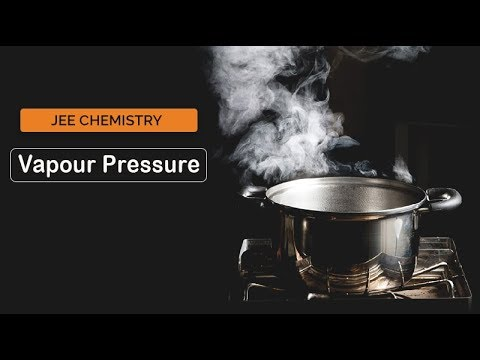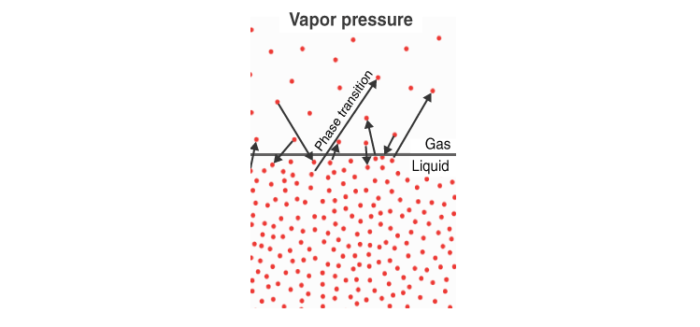# Vapour Pressure

## What is Vapour Pressure?

When a liquid is placed in a vessel that gets continually heated, the molecules of the liquid are seen to be moving at varying speeds in different directions. This happens due to the different kinetic energies possessed by the molecules of the liquid.

When the liquid is heated, the energy of the molecules rises; it becomes lighter and occupies the surface of the liquid. This process is known as ‘evaporation’. The molecules which can be seen on the liquid surface are called ‘vapor’.

The evaporation continues at a constant rate the temperature of the liquid is kept constant. When some molecules of the liquid in the vapour phase, strikes the walls of the containers or the surface of the liquid, it may get converted back to the liquid phase. This process is called condensation.

Vapour pressure, also known as vapour equilibrium pressure, can be defined as the pressure exerted (in a system featuring thermodynamic equilibrium) by a vapour with its condensed phases (solid or liquid) in a closed system at a given temperature. The equilibrium vapour pressure is known to serve as an indicator of the evaporation rate of a liquid. The propensity of particles to escape from the liquid (or a solid) is known to be related. A material that, at normal temperatures, has a high vapour pressure is generally referred to as a volatile material. It can be noted that the pressure exhibited above a liquid surface by the vapour is called vapour pressure.

## Recommended Videos## Characteristics of Vapour Pressure

However, as time passes, the number of molecules in the vapour phase increases while the rate of condensation also increases. It reaches a stage where the rate of evaporation is equal to the rate of condensation. This phase is called the stage of equilibrium.

As represented by the manometer, at this point the pressure exerted by the molecules is called the vapour pressure of the liquid. Vapour pressure is defined as the pressure exerted by the vapour present above the liquid.

Temperature is the sole factor that affects vapour pressure. The vapour pressure of a liquid is independent of the volume of liquid in the container, whether one litre or thirty litres; both samples will have the same vapour pressure at the same temperature. Temperature has an exponential connection with vapour pressure, which means that as the temperature rises, the vapour pressure rises as well.The process of evaporation depends on different factors:-

### 1. Nature of the liquid

Liquids have weak intermolecular forces. Heating the molecules of the liquid can help change them to the vapour phase and thus increase the vapour pressure of the liquid. For example, Acetone and benzene have higher vapour pressure than water at a particular temperature.

### 2. Effect of temperature

The vapour pressure of the liquid increases with an increase in its temperature. The molecules of the liquid have higher energy at higher temperatures.

## Raoult’s law

P1 ∝ x1

P1 = P1o x1,……………………………. (1)

Where P1o is the vapour pressure of component 1 in a pure state.

Similarly, for component 2:

P2 = P2o x2, …………………………….. (2)

From Dalton’s Law of partial pressures we know that,

Ptotal = P1+P2

Using the values of P1 and P2 from equation (1) and (2) respectively we have:

Ptotal = P1ox1 + P2ox2

=> Ptotal = P1o(1-x2) + P2ox2

=> Ptotal = P1o + (P2o-P1o)x2

We can draw the following inferences from the above equation:

• The above equation is a straight line between Ptotal and x2 whose slope is given by (P2o-P1o) and the y-intercept is equal to P1o.
• The total vapour pressure above a solution varies linearly with the mole fraction of component 2.
• The total vapour pressure above a solution depends on the vapour pressure of Component 1 and 2 in their pure state and the mole fraction of component 2 in the solution.

## What is a boiling point?

As we go on increasing the temperature of the liquid, its vapour pressure increases proportionately. It reaches a stage where the vapour pressure of the liquid becomes equal to the atmospheric pressure. At this temperature, the vapours near the surface start escaping to the atmosphere and the liquid undergoes a phase transition. This temperature is defined as the boiling point of the liquid.

The standard boiling point of the liquid is given at:

Pressure of 1 atm = 102325 Pa or 1 bar = 105 Pa

## What is the Heat of Vaporization?

As we provide heat to a liquid, its energy increases, which results in an increase in the overall temperature. At the boiling point, the additional heat is used up by the molecules to overcome the intermolecular force of attraction in the liquid and change to the gaseous state.

When 1 mole of liquid is transformed into a gaseous state, the amount of heat provided by this process is known as the Heat of Vaporization.

## Frequently Asked Questions – FAQs

### What is vapour pressure?

A liquid’s vapour pressure is a vapour’s equilibrium pressure above its liquid (or solid); that is, the vapour pressure resulting from a liquid (or solid) evaporation above a liquid (or solid) sample in a closed container.

### What is Raoult’s Law equation?

Raoult’s law is a chemical law that relates the solution’s vapour pressure to the mole fraction of a solution added. The law of Raoult is expressed through the formula.
Psolution = ΧsolventP0solvent.

### Is vapour pressure proportional to temperature?

When a liquid’s vapour pressure is the same as the atmospheric pressure, the material is at temperature and pressure at the boiling/freezing point. Vapour stress depends on temperature. Raoult’s law states that the solution’s vapour pressure is directly proportional to the solvent’s mole fraction.

### Does vapour pressure increase with a boiling point?

As a result, there are lower boiling points of liquids with high vapour pressure. By heating a liquid and allowing more molecules to enter the atmosphere, vapour pressure may be increased. This begins at the point where the vapour pressure is equal to the boiling atmospheric pressure.

### Which has maximum Vapour pressure?

The material with the lowest boiling point would, therefore, have the highest vapour pressure at room temperature (the easiest way to reach the gas phase). The highest boiling point material will have the lowest vapour pressure. Vapour pressure is an evaporation-related fluid element.